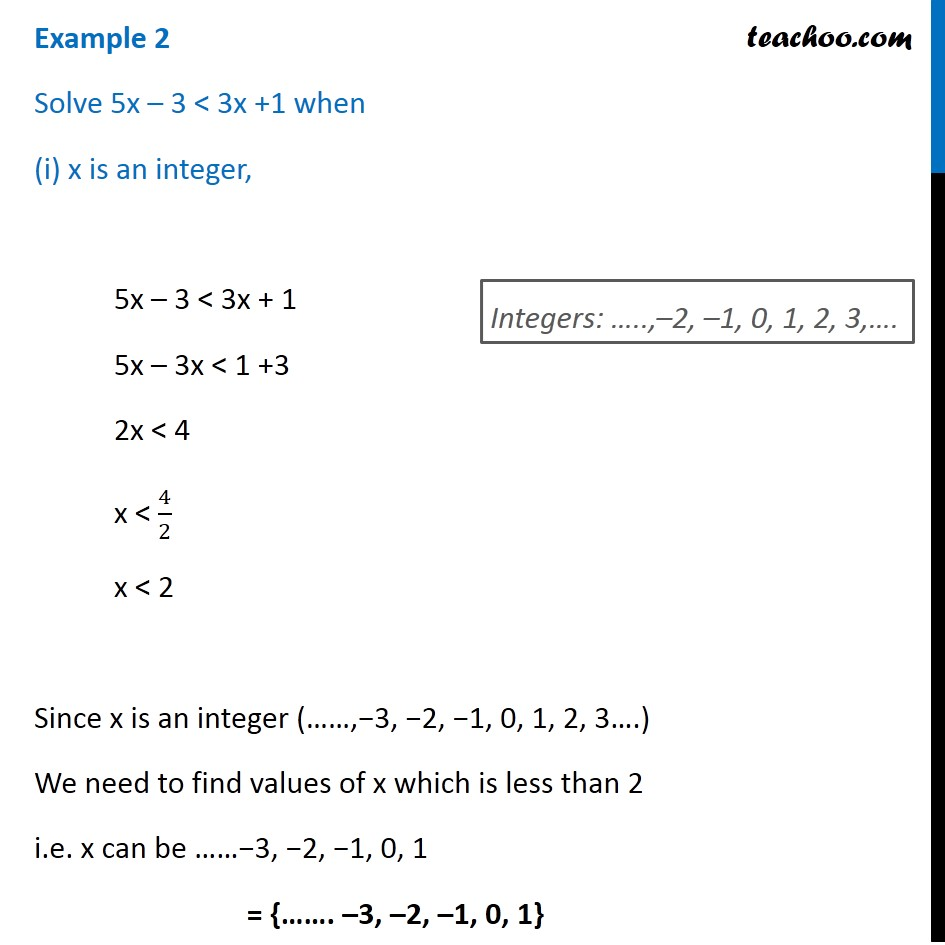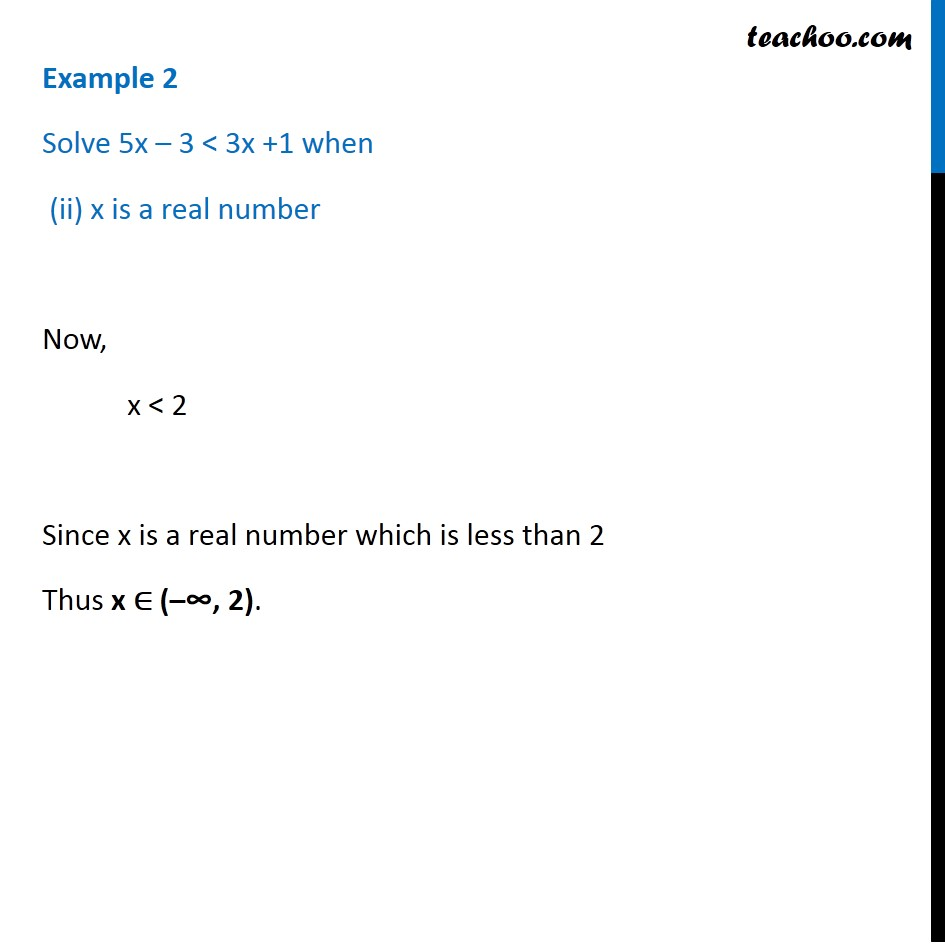Examples

Chapter 6 Class 11 Linear Inequalities
Serial order wiseMaths Crash Course - Live lectures + all videos + Real time Doubt solving!

### Transcript

Example 2 Solve 5x – 3 < 3x +1 when (i) x is an integer, 5x – 3 < 3x + 1 5x – 3x < 1 +3 2x < 4 x < 4/2 x < 2 Since x is an integer (……,−3, −2, −1, 0, 1, 2, 3….) We need to find values of x which is less than 2 i.e. x can be ……−3, −2, −1, 0, 1 = {……. –3, –2, –1, 0, 1} Integers: …..,–2, –1, 0, 1, 2, 3,…. Example 2 Solve 5x – 3 < 3x +1 when (ii) x is a real number Now, x < 2 Since x is a real number which is less than 2 Thus x ∈ (–∞, 2).